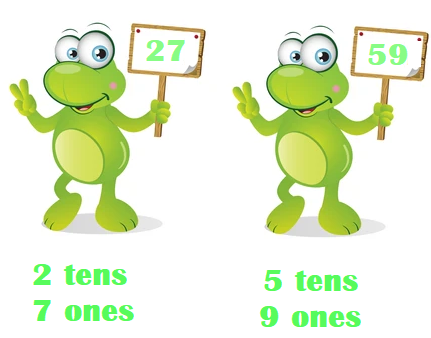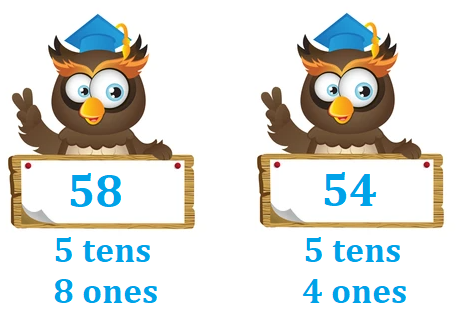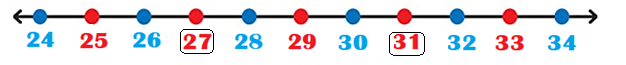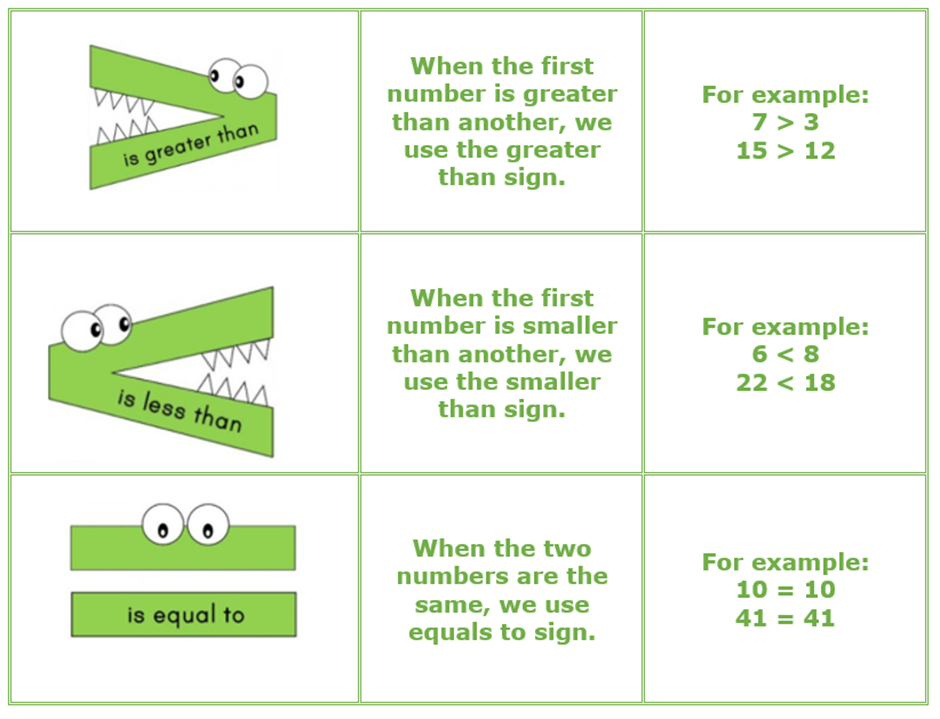# Math > Year 2

## Comparing and ordering numbers up to 100

What is Comparing?
Comparing means to know if one number is the same as, or smaller than, or bigger than, another number.

How do we compare numbers?
To compare numbers, we look at the value of digits.

For example,We start comparing numbers from the highest value column i.e., from the left-hand side.

27 have 2 tens and 59 have 5 tens.

So, 59 is bigger than 27.

What if the tens are the same?
If the tens are the same, we’ll compare the next value column i.e., ‘ones’.58 and 54 have 5 tens each. So, we’ll look at the ones digits now.
58 has ‘8 ones and 54 has ‘4 ones.
So, 58 is bigger than 54.

Comparing numbers using a number line

We can also use number lines to compare two numbers.

For example,The number on the right side of a number line is always bigger than the number on the left side.
So, 31 is bigger than 27.

Symbols
We use 3 symbols to compare numbers.What is Ordering?
Ordering means arranging in a sequence.

There are two ways of ordering:

• Ascending: Smallest to biggest
• Descending: Biggest to Smallest

Example: Order the numbers 28, 83, and 75 in ascending and descending order.

21 has 2 tens

83 has 8 tens

75 has 7 tens

Ascending order i.e., smallest to biggest is 21, 75, 83
Descending order i.e., biggest to smallest is 83, 75, 21

//